EENS 2110 Mineralogy Tulane University Prof. Stephen A. Nelson Axial Ratios, Parameters, Miller Indices

 We've now seen how crystallographic axes can be defined for the various crystal systems.  Two important points to remember are that The lengths of the crystallographic axes are controlled by the dimensions of the unit cell upon which the crystal is based. The angles between the crystallographic axes are controlled by the shape of the unit cell. We also noted last time that the relative lengths of the crystallographic axes control the angular relationships between crystal faces.  This is true because crystal faces can only develop along lattice points.  The relative lengths of the crystallographic axes are called axial ratios, our first topic of discussion. Axial Ratios Axial ratios are defined as the relative lengths of the crystallographic axes.  They are normally taken as relative to the length of the b crystallographic axis.  Thus, an axial ratio is defined as follows: Axial Ratio =  a/b : b/b : c/b where a is the actual length of the a crystallographic axis, b, is the actual length of the b crystallographic axis, and c is the actual length of the c crystallographic axis. For Triclinic, Monoclinic, and Orthorhombic crystals, where the lengths of the three axes are different, this reduces to a/b : 1 : c/b (this is usually shortened to a : 1 : c) For Tetragonal crystals where the length of the a and b axes are equal, this reduces to  1 : 1 : c/b (this is usually shorted to 1 : c) For Isometric crystals where the length of the a, b, and c axes are equal this becomes 1 : 1 : 1 (this is usually shorted to 1) For Hexagonal crystals where there are three equal length axes (a1, a2, and a3) perpendicular to the c axis this becomes: 1 : 1 : 1: c/a (usually shortened to 1 : c)  Modern crystallographers can use x-rays to determine the size of the unit cell, and thus can determine the absolute value of the crystallographic axes.  For example, the mineral quartz is hexagonal, with the following unit cell dimensions as determined by x-ray crystallography: a1 = a2 = a3 = 4.913Å c = 5.405Å where Å stands for Angstroms = 10-10 meter. Thus the axial ratio for quartz is 1 : 1 : 1 : 5.405/4.913 or 1: 1 : 1 : 1.1001 which simply says that the c axis is 1.1001 times longer than the a axes. For orthorhombic sulfur the unit cell dimensions as measured by x-rays are: a  = 10.47Å b = 12.87Å c = 24.39Å Thus, the axial ratio for orthorhombic sulfur is: 10.47/12.87 : 12.87/12.87 : 24.39/12.87 or 0.813 : 1 : 1.903 Because crystal faces develop along lattice points, the angular relationship between faces must depend on the relative lengths of the axes.  Long before x-rays were invented and absolute unit cell dimensions could be obtained, crystallographers were able to determine the axial ratios of minerals by determining the angles between crystal faces.  So, for example, in 1896 the axial ratios  of orthorhombic sulfur were determined to be nearly exactly the same as those reported above from x-ray measurements.  In a later lecture we will see how we can determine axial ratios from the angular relationships between faces.  First, however we must determine how we can name, or index faces of crystals and define directions within crystals.
 Intercepts of Crystal Faces (Weiss Parameters) Crystal faces can be defined by their intercepts on the crystallographic axes. For non-hexagonal crystals, there are three cases.
 A crystal face intersects only one of the crystallographic axes.   As an example the top crystal face shown here intersects the c axis but does not intersect the a or b axes.  If we assume that the face intercepts the c axis at a distance of 1 unit length, then the intercepts, sometimes called Weiss Parameters, are: ¥a, ¥b, 1cA crystal face intersects two of the crystallographic axes. As an example, the darker crystal face shown here intersects the a and b axes, but not the c axis.  Assuming the face intercepts the a and c axes at 1 unit cell length on each, the parameters for this face are: 1a, 1b, ¥cA crystal face that intersects all 3 axes. In this example the darker face is assumed to intersect the a, b, and c crystallographic axes at one unit length on each.  Thus, the parameters in this example would be:  1a, 1b, 1c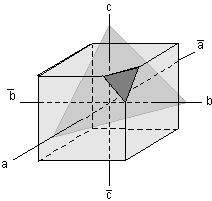Two very important points about intercepts of faces: The intercepts or parameters are relative values, and do not indicate any actual cutting lengths.   Since they are relative, a face can be moved parallel to itself without changing its relative intercepts or parameters. Because one does usually not know the dimensions of the unit cell, it is difficult to know what number to give the intercept of a face, unless one face is chosen arbitrarily to have intercepts of 1.  Thus, the convention is to assign the largest face that intersects all 3 crystallographic axes the parameters  - 1a, 1b, 1c.  This face is called the unit face.
 For example, in the orthorhombic crystal shown here, the large dark shaded face is the largest face that cuts all three axes.  It is the unit face, and is therefore assigned the parameters 1a, 1b, 1c.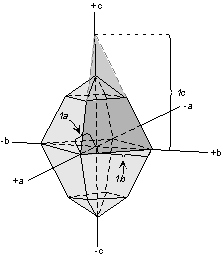Once the unit face is defined, the intercepts of the smaller face can be determined.  These are 2a, 2b, 2/3c.  Note that we can divide these parameters by the common factor 2, resulting in 1a,1b,1/3c. Again, this illustrates the point that moving a face parallel to itself does not change the relative intercepts.  Since intercepts or parameters are relative, they do not represent the actual cutting lengths on the axes.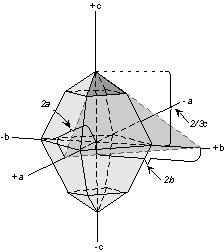By specifying the intercepts or parameters of a crystal face, we now have a way to uniquely identify each face of a crystal.  But, the notation is cumbersome, so crystallographers have developed another way of identifying or indexing faces.  This conventional notation called the Miller Index is our next topic of discussion.
 Miller Indices The Miller Index for a crystal face is found by first determining the parameters second inverting the parameters, and third clearing the fractions. For example, if the face has the parameters 1 a, 1 b,  ¥ c inverting the parameters would be 1/1, 1/1, 1/ ¥ this would become 1, 1, 0 the Miller Index is written inside parentheses with no commas - thus (110)
 As further examples, let's look at the crystal shown here.  All of the faces on this crystal are relatively simple.  The face [labeled (111)] that cuts all three axes at 1 unit length has the parameters 1a, 1b, 1c.  Inverting these, results in 1/1, 1/1, 1/1 to give the Miller Index (111).   The square face that cuts the positive a axis, has the parameters 1 a, ¥ b, ¥ c.  Inverting these becomes 1/1, 1/¥, 1/¥ to give the Miller Index (100). The face on the back of the crystal that cuts the negative a axis has the parameters -1a, ¥ b, ¥ c.  So its Miller Index is (00).  Note how the negative intercept is indicated by putting a minus sign above the index.  This would be read "minus one, one, one".  Thus, the other 4 faces seen on this crystal would have the Miller Indices (001),  (00), (010), and (00).Now let's look at some more complicated examples.  The drawing to the right is the same orthorhombic crystal we looked at earlier.  Recall that the small triangular face near the top that cuts all three axes had the parameters 1a, 1b, 1/3c.   Inverting these becomes 1/1, 1/1, 3/1 to give the Miller Index for this face as (113). Similarly, the small triangular face the cuts the positive a axis and the negative b axis, would have the Miller Index (13), the similar face on the bottom of the crystal, cutting positive a, positive b, and negative c axes would have the Miller Index   (11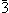).   See if you can determine the Miller Indices for the 8 faces on the back of the crystal that are not seen in this drawing.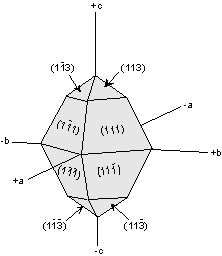Note once again, that moving a face parallel to itself does not change the parameters nor the Miller Index for that face. To refer to a general face that intersects all three crystallographic axes where the parameters are not known, we use the notation (hkl).  For a face that intersects the b and c axes with general or unknown intercepts the notation would be (0kl),  for a face intersecting the a and c axis, but parallel to b the notation would be (h0l), and similarly for a face intersecting the a and b axes, but parallel to c we would use the notation (hk0).
 This Miller Index notation applies very well to crystals in the Triclinic, Monoclinic, Orthorhombic, Tetragonal, and Isometric systems,  but requires some modification to be applied to the Hexagonal crystal system.   Miller Bravais Indices Since the hexagonal system has three "a" axes perpendicular to the "c" axis, both the parameters of a face and the Miller Index notation must be modified.  The modified parameters and Miller Indices must reflect the presence of an additional axis.  This modified notation is referred to as Miller-Bravais Indices, with the general notation (hkil)
 To see how this works, let's look at the dark shaded face in the hexagonal crystal shown here.  This face intersects the positive a1 axis at 1 unit length, the negative a3 axis at 1 unit length, and does not intersect the a2 or c axes.  This face thus has the parameters: 1 a1,   ¥ a2, -1 a3,  ¥ c Inverting and clearing fractions gives the Miller-Bravais Index: (100) An important rule to remember in applying this notation in the hexagonal system, is that whatever indices are determined for h, k, and i,  h + k + i = 0For a similar hexagonal crystal, this time with the shaded face cutting all three axes, we would find for the shaded face in the diagram that the parameters are 1 a1, 1 a2, -1/2 a3,  ¥ c.  Inverting these intercepts gives: 1/1, 1/1, -2/1, 1/¥ resulting in a Miller-Bravais Index of  (110) Note how the "h + k + i = 0" rule applies here!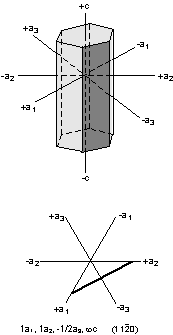Crystal Forms Although we will not cover this in detail in this lecture, the next step is to use the Miller Index notation to designate crystal forms.  A crystal form is a set of crystal faces that are related to each other by symmetry.  To designate a crystal form (which could imply many faces) we use the Miller Index, or Miller-Bravais Index notation enclosing the indices in curly braces, i.e. {hkl} or {hkil} Such notation is called a form symbol.
 As an example, look at the crystal drawing shown here.  This crystal is the same orthorhombic crystal discussed above.  It has two forms.  The form {111} consists of the following symmetrically  8 related faces: (111),  (11), (11), (1), (11), (1), (1), and ().   This form is called a rhombic-dipyramid. The other form is also a rhombic-dipyramid, but consists of the triangular shaped faces similar to the face (113).  The form symbol for this form is {113} and consists of the following 8 faces: (113), (13), (11), (1), (13), (1), (3), and ().   We'll go over this in more detail in the next lecture.Examples of questions on this material that could be asked on an exam Define the following: (a) axial ratio, (b) unit face, (c) crystal form, d) form symbol, (3) Miller Index Explain why a crystal face can be moved parallel to itself without changing its Miller Index What is the importance of Miller Indices in crystallography? Return to EENS 2110 Page